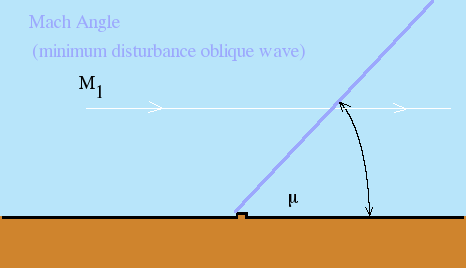Mach Angle Calculations

This form calculates the Mach Angle for a supersonic air flow. This is the angle of the minimal oblique wave that is created by a small disturbance in the air flow. The required input is the Mach number of the upstream flow. The gas is assumed to be ideal air.

Tabulated DataInputs
 Upstream Mach Number (M1)

Results
 Mach Angle (mu)(deg)

(c) 2006 AMME, University of Sydney.